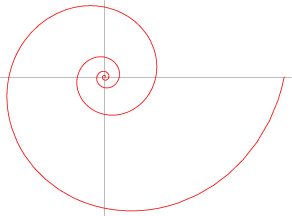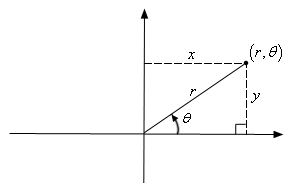JavaScript 数学曲线—等角螺线

简介• r ：与原点的距离。

• a ：常数。

• b ：常数。

• e ：常数。

• θ ：与 x 轴的角度。

• 鹦鹉螺的贝壳像等角螺线。

• 菊的种子排列成等角螺线。

• 昆虫以等角螺线的方式接近光源。

• 旋涡星系的旋臂差不多是等角螺线。

• 低气压(热带气旋、温带气旋等)的外观像等角螺线

绘制x = rcos(θ)

y = rsin(θ)

θ = arctan(y/x)function draw() {

let a = 0.1, b = 0.3, angle = 0;

let x = 0, y = 0, points = [];

const acceleration = 0.1, circleNum = 4;

// 注意这里角度的递增，以 2 * Math.PI 为基准进行比较，控制画多少圈

while (angle <= circleNum * 2 * Math.PI) {

const anglePow = Math.pow(Math.E, b * angle);

x = a * anglePow * Math.cos(angle);

y = a * anglePow * Math.sin(angle);

points.push([x, y]);

angle = angle + acceleration;

}

// 实现把点绘制成线的方法

line({ points: points});

}

参考资料